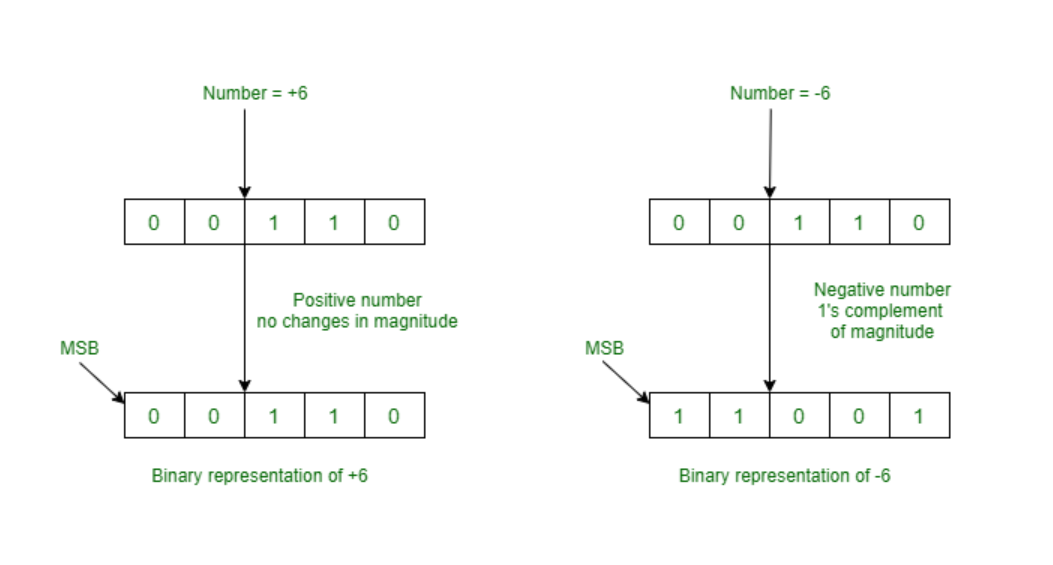Open in App
Not now

# One’s Complement

• Difficulty Level : Basic
• Last Updated : 05 Oct, 2022

In digital electronics, the binary system is one of the most common number representation techniques. As its name suggest binary number system deals with only two number 0 and 1, this can be used by any device which is operating in two states. The binary number system has two complements 1’s and 2’s complement.

### One’s Complement:

In simple words, if we want to understand the One’s complement, so one’s complement is toggling or exchanging all the 0’s into 1 and all the 1’s into 0 of any number. Suppose there is a binary number 11001001, then its one’s complement will be 00110110.

In actuality, the one’s complement means the addition of a negative integer to the number, and this eliminates the requirement of a separate subtraction processor.

### How to Find One’s Complement of a Number:

To find one’s complement of any number, follow the below steps:

1. Convert the number of any number system to a binary number system, i.e. if the number is in octal, decimal, hexadecimal, or any other number system; so convert it into a binary number system. If there is a number 10 and we need to find its one complement convert it into a binary number system, i.e. 1010.
2. After, having the number in binary form. Now, invert or exchange all the 0’s to 1 and all the 1’s to 0. Like, 1010 will be 0101.
3. The resulting binary number is the 1’s complement of the given number.

CASE 1: If the decimal number was a negative number, suppose we have a number say -10. To find out 1’s complement of such number. Follow the given steps:

1. Convert the number into the binary number system as we do for the positive number.
2. Now, exchange all the 1’s to 0 and 0’s to 1.
3. Take the transpose of the negative binary number.

Examples:

1. Evaluate the 1’s complement of the 11010011.

Solution: Simply invert each bit of the number, and hence the 1’s complement for the above binary number is – 00101100

2. Evaluate the 1’s complement for the fractional binary number 00111.001.

Solution: The 1’s complement representation of the fractional binary number will be 11000.110.

3. Evaluate 1’s complement of all 4-bit binary numbers

### 1’s complement representation in Signed Magnitude:

In sign-magnitude, the leftmost bit indicates the sign of the integer. Representation of the positive remains the same. In sign-magnitude, 6 is a positive number, its binary representation is 0000 0110, and it will be represented as it is, i.e. 0000 0110. Whereas a negative number suppose -6 will be represented as 1111 1001. Negative integers are generated by reversing all the bits or by performing a bitwise complement of a positive integer.### Range of 1’s Complement Number:

For n bits register, the smallest negative number that can be stored is -(2(n-1)-1) and the largest positive largest number that can be stored is (2(n-1)-1).

NOTE: This (sign) representation has an ambiguous representation of the number 0. It has two different representations for 0; negative zero (-0) is 1 1111 in the five-bit register and the positive zero (+0) is 0 0000 in the five-bit register.

### Application of 1’s Complement:

• Represent Signed binary number: 1’s complement is used to represent signed binary numbers, as positive signed binary numbers can be represented as it is, 1’s complement is widely used to represent negative binary numbers in sign-magnitude.
• 1’s complement is also used in various arithmetic binary operations like subtraction, addition, etc.

For more details, you can refer  Difference between 1’s Complement representation and 2’s Complement representation Technique article.

My Personal Notes arrow_drop_up